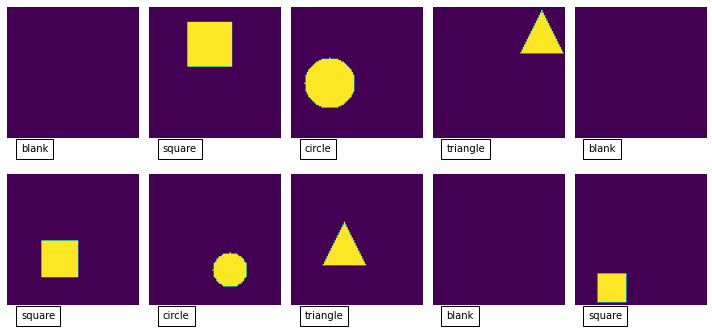Article Image
Article Image

Categorical classification is used where you have data that falls into one of many categories - an example of this would be classifying food - e.g. “hot dog”, “pizza”, “fries” etc…

You can find the example notebook(s) for this post in the tensorflow-tutorial GitHub repo.

For this small tutorial, I’ve created a python generator that creates images that are either blank, a square, a circle, or a triangle.def data_generator():
i = 0
while(True):
if i >= 1000:
i = 0
# our output value will be the one hot encoded version of: 0,1,2,3 - corresponding to our labels - "blank", "square", "circle", "triangle"
Y = i % 4
X = np.zeros((image_width, image_height, 1))

# size of our shape
# position of our shape

if Y == 1: # generate a square
elif Y == 2: # generate a circle
X[y+center_y, x+center_x] = 1
elif Y==3:
X[y+center_y, x+center_x] = 1
else: # blank image
pass
yield X, tf.one_hot(Y, 4)
i = i + 1


We are now using one-hot encoding for our label:

        yield X, tf.one_hot(Y, 4)


For our four labels this will result in the following labels:

blank =    [1, 0, 0, 0]
square =   [0, 1, 0, 0]
circle =   [0, 0, 1, 0]
triangle = [0, 0, 0, 1]


To get categorical classification working we need to take note of a couple of things:

1. We need to have the same number of output neurons as our classes - in this case four. We also need to use the softmax activation function. The softmax activation function will make sure the total output from all our neurons sum to 1. We can then use the output from each one as the probability that the input belongs to that class.
2. We need to use the (CategoricalCrossentropy)[https://www.tensorflow.org/api_docs/python/tf/keras/losses/CategoricalCrossentropy] loss function during our training.

Our simple model looks like this:

model = Sequential([
Conv2D(8, 3,
activation='relu',
input_shape=(image_width, image_height, 1),
name='conv_layer'),
MaxPooling2D(name='max_pooling'),
Flatten(),
Dense(
10,
activation='relu',
name='hidden_layer'
),
Dense(4, activation='softmax', name='output')
])


And when we compile it we specify the loss function that we want to optimise:

model.compile(optimizer='adam',
loss=tf.keras.losses.CategoricalCrossentropy(),
metrics=['accuracy'])


This is a slightly more complex problem that the binary classification problem from this post so we need to train for more epochs to get 100% accuracy, but you should reach this in about 10 epochs.

You can test the model pretty easily by feeding in some more random samples from the training set:

# get a batch of samples from the dataset
X, _ = next(iter(train_dataset))
# ask the model to predict the output for our samples
predicted_Y = model.predict(X.numpy())
# work out the max indices
max_indices = tf.argmax(predicted_Y, axis = 1)
# show the images along with the predicted value
plot_images(X, max_indices)
# set the format to 2 decimal places
np.set_printoptions(formatter={'float': lambda x: "{0:0.2f}".format(x)})
predicted_Y[:10]


If we look at the output from this:You can see that it is pretty good at predicting the different categories that our images fall into:

array([[1.00, 0.00, 0.00, 0.00],
[0.00, 1.00, 0.00, 0.00],
[0.00, 0.00, 1.00, 0.00],
[0.00, 0.00, 0.05, 0.95],
[1.00, 0.00, 0.00, 0.00],
[0.00, 1.00, 0.00, 0.00],
[0.00, 0.00, 1.00, 0.00],
[0.00, 0.00, 0.01, 0.99],
[1.00, 0.00, 0.00, 0.00],
[0.00, 0.96, 0.04, 0.00]], dtype=float32)


Checkout the full code in the GitHub repo.

> Image